# Simi

•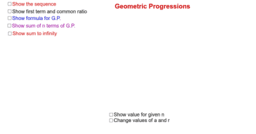### Geometric Progression

Activity

Simi

•### Arithmetic Progressions

Activity

Simi

•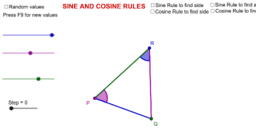### Sine and Cosine Rules

Activity

Simi

•### SOHCAHTOA/Pythagoras

Activity

Simi

•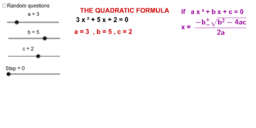Activity

Simi

•Activity

Simi

•Activity

Simi

•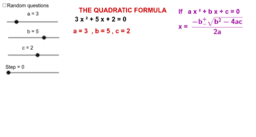Activity

Simi

•### Equation of a Line

Activity

Simi

•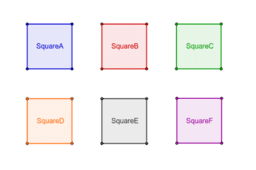### Squares, Squares, Squares,...

Activity

Simi

•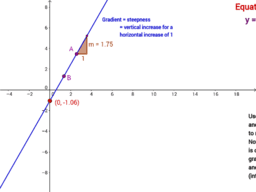### Equation of a straight line

Activity

Simi

•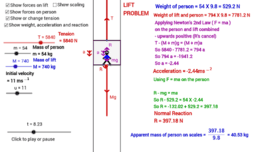### The Lift Problem - Mechanics

Activity

Simi

•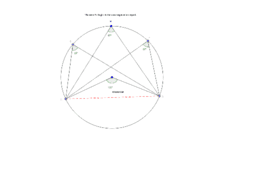Activity

Simi